Relativity and Cosmology Blog# Relativity and Cosmology

This is a Blog on relativity and cosmology for engineers and the like. My website "Relativity-4-Engineers" has more in-depth stuff.

Comments/questions of a general nature should preferably be posted to the FAQ section of this Blog (http://cr4.globalspec.com/blogentry/316/Relativity-Cosmology-FAQ).

A complete index to the Relativity and Cosmology Blog can be viewed here: http://cr4.globalspec.com/blog/browse/22/Relativity-and-Cosmology"

Regards, Jorrie

 Previous in Blog: SpaceProperTime Diagrams and the Central Singularity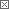# Pesky Ellipse Circumference

Posted October 04, 2020 6:10 AM by Jorrie
Pathfinder Tags: circumference ellipse perimeter

When I first heard that there is no easy exact formula for the circumference of the standard ellipse, I found it hard to believe. I had already learned that a planet traces out an ellipse with the Sun at one of the foci. And that the orbital period is proportional to the three-halves power of the semi-major axis of the orbit,where:

• a: semi-major axis,
• b: semi-minor axis.

This is Kepler's third law of planetary motion. It gives this easy equation for the period of the orbit:where

This ignores relativistic effects, of course, so the orbit is and exact ellipse.

If the elliptical period is so simple, then why is the circumference of the orbit a problem? After all, Kepler's second orbital law also states that the planet sweeps out a constant area per unit time, as observed in Sun-centered coordinates.

This is the consequence of the constancy (conservation) of angular momentum around an orbit, so for each position on the ellipse perimeter, it is trivial to calculate the orbital velocity. So we have velocity and we have time - the distance that the planet travel should also be trivial. Or not?

The precise value for the perimeter length (circumference) is given by the following infinite series: (Wikipedia Ellipse Circumference)where !! is the double factorial.

This series converges, but by expanding in terms of

h = ( a − b ) 2 / ( a + b ) 2 ,

James Ivory and Bessel derived an expression that converges much more rapidly:I leave you with the puzzle: why is the period and the velocity of the elliptical orbit trivial, but the precise perimeter length so complex?

Interested in this topic? By joining CR4 you can "subscribe" to
Guru

Join Date: Apr 2010
Location: About 4000 miles from the center of the earth (+/-100 mi)
Posts: 9249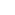#1

###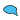Re: Pesky Ellipse Circumference

10/04/2020 10:43 AM

Yeah, you would think you could just start with the formula for the circle perimeter (integral r dΘ), and replace dΘ with a foreshortened value based on the angle Θ and eccentricity e = b/a.

Alas, it seems some functions are not meant to be integrated analytically. Fortunately, you can computer integrate it nowadays, making dΘ as small as need be.

Guru

Join Date: Apr 2010
Location: About 4000 miles from the center of the earth (+/-100 mi)
Posts: 9249#4

###Re: Pesky Ellipse Circumference

10/04/2020 2:50 PM

A few details:

Ellipse is dimensions 2a x 2b (a>b)

given major and minor axes a and b, calculate eccentricity esince circle circumference is C = ∫02pi r dθ, when you account for the eccentricity (e) and position on the circle (θ) of the incremental segment dθ, the dθ foreshortened length will be sqrt(1-e2sin2(θ))dθ, a replaces r, and because of symmetry, you only have to compute 1/4 of the ellipse and multiply by 4.A computer program would calculate:

function circum = EllipseCircumference (a, b, dtheta)

## a = major axis (radius)

## b = minor axis (radius)

## dtheta = angle increment size (radians)

## circum = circumference

e=sqrt(1-(b/a)^2);

circum=0;

theta=0;

while theta < pi/2

circum = circum + 4*a*sqrt(1-(e^2)*sin(theta)^2)*dtheta;

theta = theta + dtheta;

endwhile

endfunction#6

###Re: Pesky Ellipse Circumference

10/05/2020 1:23 AM

Yes, numerical integration is always a way out, but the penalty paid is the effort to implement an algorithm like the one that you proposed. This will give an answer equivalent to the infinite series, both depending on how many steps are calculated.

From an engineering perspective, approximations of 1% or better are normally good enough for all practical purposes. And it would be nice to have a more straightforward equation to approximate it with, one that you can easily write into your documentation.

There are many, but my favorite one was derived by Srinivasa Ramanujan, a brilliant early-1900's mathematician. He somehow came up with a simple, better than 0.1% empirical equation. It is good for b:a ratios of more than 5, so it works for planet Mercury with good accuracy.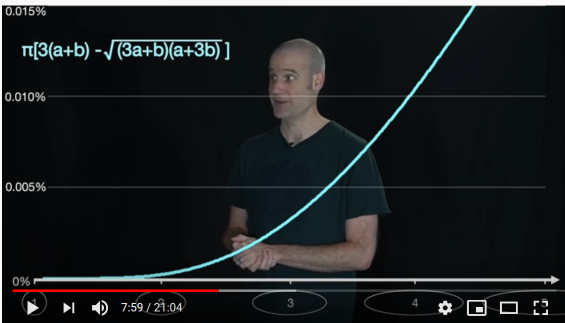Nicely symmetrical and clean!

__________________
"Perplexity is the beginning of knowledge." -- Kahlil Gibran
Guru

Join Date: Apr 2010
Location: About 4000 miles from the center of the earth (+/-100 mi)
Posts: 9249#7

###Re: Pesky Ellipse Circumference

10/06/2020 11:41 AM

Ramanujan, with little formal education (and probably because of it), was able to solve mathematical problems that everyone else "knew" were impossible.His formula is much faster than integrating your way around the ellipse, especially taking "baby steps" for more accuracy.

Guru

Join Date: Mar 2007
Location: by the beach in Florida
Posts: 31843#2

###Re: Pesky Ellipse Circumference

10/04/2020 12:49 PM

It seems like you could make a formula using a series of the radii of a quarter section....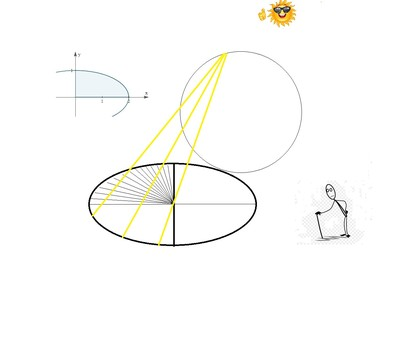After all it is a circle viewed from a specific angle...

__________________
All living things seek to control their own destiny....this is the purpose of life
Guru

Join Date: Mar 2007
Location: by the beach in Florida
Posts: 31843#3

###Re: Pesky Ellipse Circumference

10/04/2020 2:38 PM
__________________
All living things seek to control their own destiny....this is the purpose of life
Guru

Join Date: Mar 2007
Location: by the beach in Florida
Posts: 31843#5

###Re: Pesky Ellipse Circumference

10/04/2020 3:47 PM

__________________
All living things seek to control their own destiny....this is the purpose of life
Power-User

Join Date: Feb 2020
Posts: 260#8

###Re: Pesky Ellipse Circumference

11/30/2020 12:03 PM

There might be an easier way. Whenever you see a ellipse in nature, think of a one turn closed(circular) helix. Subtract the perigee from the apogee. This result will be the radius of the one turn helix. Think of a one turn stripe on a barber pole.....then bend pole into a circle. The real circumference is the length of that stripe. A non-pi circumference.
That's how nature makes an ellipse.......in other words, nature does not use an ellipse.

__________________
The proper hardware will eliminate all theory.